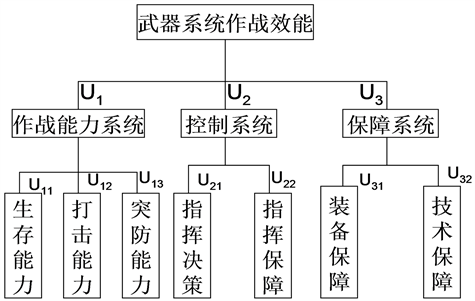﻿ 基于最大熵原理的武器作战效能预测研究

# 基于最大熵原理的武器作战效能预测研究The Prediction of Effectiveness of Weapons and Combat Based on the Principle of Maximum Entropy

Abstract: AHP and fuzzy comprehensive evaluation (FCE) were respectively used for the forecast of the ef-fectiveness of weapons and combat. Then on the basis of merit of maximum entropy principle, the traditional method has been improved, and concluded that the principle of maximum entropy had higher forecast accuracy. Thus it can play a guiding role on the actual operations.

Abstract:

1. 引言

2. 武器系统的评价指标Figure 1. Evaluation index system of weapon system effectiveness

3. 具体案例分析

3.1. AHP预测武器作战效能 

3.1.1. 构建两两比较判断矩阵A

3.1.2. 计算判断矩阵的各行元素的积的n (判断矩阵的阶数)次方根 $\stackrel{¯}{{\omega }_{i}}$

$\stackrel{¯}{{\omega }_{1}}=2.2894$$\stackrel{¯}{{\omega }_{2}}=0.3816$$\stackrel{¯}{{\omega }_{3}}=1.1447$ ，并将其归一化得到： ${\omega }_{1}=0.6000$${\omega }_{2}=0.1000$${\omega }_{3}=0.3000$

3.1.3. A的满意一致性检验

$CI=\left({\lambda }_{\mathrm{max}}-n\right)/\left(n-1\right)=0.00005$ ，其中 ${\lambda }_{\mathrm{max}}$ 为判断矩阵A的最大特征值。判断矩阵A的随机一致性指标 $CR=CI/RI<0.1$ (RI为常数)，A具有满意一致性。故U1，U2，U3的指标权重分别为0.6000，0.1000，0.3000。

3.1.4. 预测武器作战效能

EAHP = 0.6 * [0.1 0.2 0.7] * [1 10 2]’ + 0.1 * [0.3 0.7] * [2 5]’ + 0.3 * [0.7 0.3] * [5 10]’ = 4.46 (式中的整数矩阵部分可根据判断矩阵，再结合白化权函数得到)。

3.2. FCE法预测武器作战效能 

3.2.1. 确定模糊关系矩阵(根据判断矩阵和白化权函数得到)

3.2.2. 预测武器作战效能

B2 = U1 * R1 = [0.2610 0.1186 0.2004 0.2972 0.1227]，

B3 = U1 * R1 = [0.0767 0.0677 0.2947 0.3704 0.1906]。

3.3. 最大熵原理预测武器作战效能 

3.3.1. 最大熵原理

1948年，香农(Shannon)用熵来定量地描述一个随机事件的不确定性或信息量：

$H=-\underset{i=1}{\overset{n}{\sum }}{p}_{i}\mathrm{ln}{p}_{i}$ (1)

$\underset{i=1}{\overset{n}{\sum }}{p}_{i}=1$ (2)Table 2. Fuzzy relation matrix R1Table 3. Fuzzy relation matrix R2Table 4. Fuzzy relation matrix R3

${E}_{k}=\underset{i=1}{\overset{n}{\sum }}{f}_{k}\left({x}_{i}\right){p}_{i}$ ( $k=1,2,\cdots ,m$ ) (3)

$H-\alpha -\underset{k=1}{\overset{m}{\sum }}{\beta }_{k}{E}_{k}$ (4)

$H=\underset{i=1}{\overset{n}{\sum }}{p}_{i}\mathrm{ln}\left\{\frac{1}{{p}_{i}}\mathrm{exp}\left[-\alpha -\underset{k=1}{\overset{m}{\sum }}{\beta }_{k}{f}_{k}\left({x}_{i}\right)\right]\right\}$ (5)

$H\le \underset{i=1}{\overset{n}{\sum }}{p}_{i}\left\{\frac{1}{{p}_{i}}\mathrm{exp}\left[-\alpha -\underset{k=1}{\overset{m}{\sum }}{\beta }_{k}{f}_{k}\left({x}_{i}\right)\right]-1\right\}+\alpha +\underset{k=1}{\overset{m}{\sum }}{E}_{k}$ (6)

${p}_{i}=\mathrm{exp}\left[-\alpha -\underset{k=1}{\overset{m}{\sum }}{\beta }_{k}{f}_{k}\left({x}_{i}\right)\right]$ ( $i=1,\cdots ,n$ )时，H取得最大值 (7)

3.3.2. 预测武器作战效能

$\mathrm{max}H\left(x\right)=-\int p\left(x\right)\mathrm{ln}p\left(x\right)\text{d}x$ (8)

$\int p\left(x\right){\left[\left(x-\stackrel{^}{{y}_{r}}\right)/\stackrel{^}{{y}_{r}}\right]}^{2}\text{d}x={\left(\frac{y-\stackrel{^}{{y}_{r}}}{\stackrel{^}{{y}_{r}}}\right)}^{2}\text{\hspace{0.17em}}\text{\hspace{0.17em}}\left(r=1,2\right)$ (9)

$\int p\left(x\right)\text{d}x=1$ (10)

$p\left(x\right)=\mathrm{exp}\left[-\alpha -\underset{r=1}{\overset{2}{\sum }}{\beta }_{r}{\left(\frac{x-\stackrel{^}{{y}_{r}}}{\stackrel{^}{{y}_{r}}}\right)}^{2}\right]$ (11)

${\int }_{1}^{9}{\left(x-\stackrel{^}{{y}_{r}}\right)}^{2}\mathrm{exp}\left(-\alpha -{\beta }_{1}{\left(\frac{x-\stackrel{^}{{y}_{1}}}{\stackrel{^}{{y}_{1}}}\right)}^{2}-{\beta }_{2}{\left(\frac{x-\stackrel{^}{{y}_{2}}}{\stackrel{^}{{y}_{2}}}\right)}^{2}\right)\text{d}x={\left(y-\stackrel{^}{{y}_{r}}\right)}^{2}$ (12)

${\int }_{1}^{9}\mathrm{exp}\left(-\alpha -{\beta }_{1}{\left(\frac{x-\stackrel{^}{{y}_{1}}}{\stackrel{^}{{y}_{1}}}\right)}^{2}-{\beta }_{2}{\left(\frac{x-\stackrel{^}{{y}_{2}}}{\stackrel{^}{{y}_{2}}}\right)}^{2}\right)\text{d}x=1$ (13)

$\alpha =2$${\beta }_{1}=42.04$${\beta }_{2}=-31.11$ 则， $p\left(x\right)=\mathrm{exp}\left[-2-\text{42}\text{.04}*{\left(\frac{x-4.46}{4.46}\right)}^{2}+\text{31}\text{.11}*{\left(\frac{x-4.1503}{4.1503}\right)}^{2}\right]$ (14)

4. 结果分析

5. 小结

 王磊, 吴静, 李旭昌. 基于串并联模型的武器系统作战效能评估[J]. 弹箭与制导学报, 2008, 28(1): 303-306.

 陈雪娣, 刘峥, 张守宏. 目标闪烁对地空导弹杀伤效能的影响分析[J]. 弹箭与制导学报, 2006, 26(4): 143-146.

 刘勇, 武昌, 林健. 无线电导航系统作战效能评估研究[J]. 弹箭与制导学报, 2007, 27(5): 268-270.

 田棣华, 马宝华, 范宁军. 兵器科学技术总论[M]. 北京: 北京理工大学出版社, 2004.

 朱传志, 孙旭明, 马士友. 基于三角白化权函数的导弹分队信息作战能力评估[J]. 兵工自动化, 2006, 25(6): 9-10.

 马琳, 宋贵宝, 罗云宝. 导弹武器系统效能评估GAHP模型[J]. 系统仿真学报, 2006, 18(z2): 101-103.

 林天峰. 基于最大熵原理的网络流量预测综合模型[J]. 微电子学与计算机, 2006, 23(8): 147-149.

Top